# Samacheer Kalvi Books: Tamilnadu State Board Text Books Solutions

## Samacheer Kalvi 9th Maths Chapter 5 Coordinate Geometry Notes PDF Download: Tamil Nadu STD 9th Maths Chapter 5 Coordinate Geometry NotesSamacheer Kalvi 9th Maths Chapter 5 Coordinate Geometry Notes PDF Download: Tamil Nadu STD 9th Maths Chapter 5 Coordinate Geometry Notes

## Samacheer Kalvi 9th Maths Chapter 5 Coordinate Geometry Notes PDF Download

We bring to you specially curated Samacheer Kalvi 9th Maths Chapter 5 Coordinate Geometry Notes PDF which have been prepared by our subject experts after carefully following the trend of the exam in the last few years. The notes will not only serve for revision purposes, but also will have several cuts and easy methods to go about a difficult problem.

 Board Tamilnadu Board Study Material Notes Class Samacheer Kalvi 9th Maths Subject 9th Maths Chapter Chapter 5 Coordinate Geometry Format PDF Provider Samacheer Kalvi Books

## How to Download Samacheer Kalvi 9th Maths Chapter 5 Coordinate Geometry Notes PDFs?

2. Click on the Samacheer Kalvi 9th Maths Notes PDF.
3. Look for your preferred subject.
4. Now download the Samacheer Kalvi 9th Maths Chapter 5 Coordinate Geometry notes PDF.

## Tamilnadu Samacheer Kalvi 9th Maths Solutions Chapter 5 Coordinate Geometry Additional Questions

Exercise 5.1

Question 1.
State whether the following statements are true/false.
(i) (5, 7) is a point in the IV quadrant.
(ii) (-2, -7) is a point in the III quadrant.
(iii) (8, -7) lies below the x-axis.
(iv) (-2, 3) lies in the II quadrant.
(v) For any point on the x-axis its y-coordinate is zero.
Solution:
(i) False
(ii) True
(iii) True
(iv) True
(v) True

Question 2.
Locate the points
(i) (3, 5) and (5, 3)
(ii) (-2, -5) and (-5, -2) in the rectangular coordinate system.
Solution: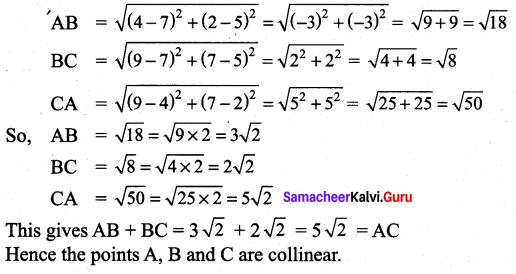Question 3.
In which quadrant does the following points lie?
(i) (5, 2)
(ii) (-5, -8)
(iii) (-7, 1)
(iv) (8, -3)
Solution:

Question 4.
Write down the ordinate of the following points.
(i) (7, 5)
(ii) (2, 9)
(iii) (-5, 8)
(iv) (7, -4)
Solution:
(i) 5
(ii) 9
(iii) 8
(iv) -4 (ordinate is the y-coordinate)

Exercise 5.2

Question 1.
Find the distance between the following pairs of points.
(i) (-4, 0) and (3, 0)
(ii) (-7, 2) and (5, 2)
Solution:
(i) The points (-4, 0) and (3, 0) lie on the x-axis. Hence,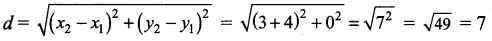(ii) The points (5,2) and (-7,2) lie on a line parallel to the x-axis. Hence the distance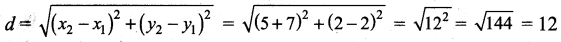Question 2.
Show that the three points (4, 2), (7, 5) and (9, 7) lie on a straight line.
Solution:
Let the points be A(4, 2), B(7, 5) and C(9, 7). By the distance formula.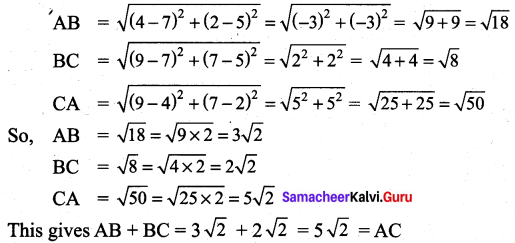Hence the points A, B and C are collinear.

Question 3.
Determine whether the points are vertices of a right triangle A(-3, -4), B(2, 6) and C (-6, 10).
Solution: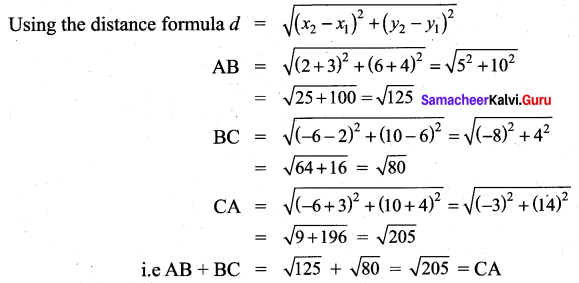Hence ABC is a right angled triangle since the square of one side is equal to sum of the squares of the other two sides.

Question 4.
Show that the points (a, a), (-a, -a) and (−a3–√,a3–√) form an equilateral triangle.
Solution:
Let the points be represented by A (a, a), B(-a, -a) and C(−a3–√,a3–√) using the distance formula.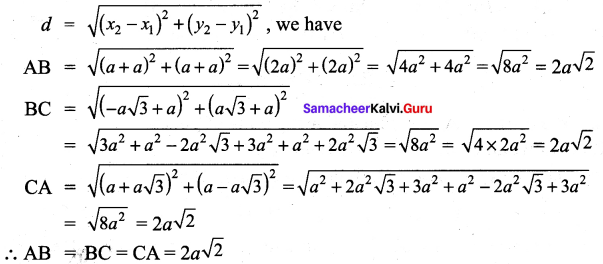Since all the sides are equal the points form an equilateral triangle.

Question 5.
Prove that the points (-7, -3), (5, 10), (15, 8) and (3, -5) taken in order are the corners of a parallelogram.
Solution:
Let A, B, C and D represent the points (-7, -3), (5, 10), (15, 8) and (3, -5) respectively.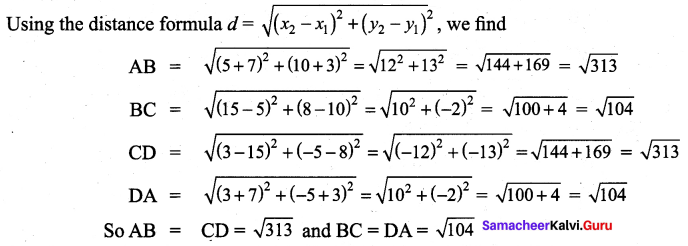i.e. The opposite sides are equal. Hence ABCD is a parallelogram.

Question 6.
Show that the following points A (3, 1) B(6, 4) and C(8, 6) lies on a straight line.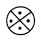Solution:
Using the distance formula, we have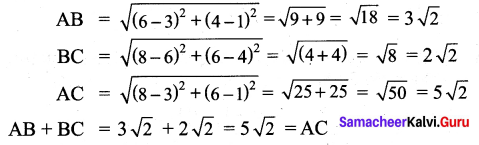Therefore the points lie on a straight line.

Question 7.
If the distance between the points (5, -2), (1, a) is 5 units. Find the value of a.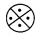Solution: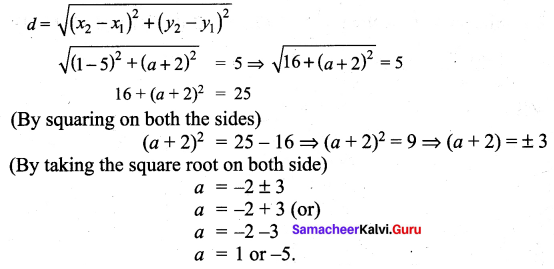Exercise 5.3

Question 1.
A, B and C are vertices of ∆ ABC. D, E and F are mid points of sides AB, BC and AC respectively. If the coordinates of A, D and F are (-3, 5), (5, 1) and (-5, -1) respectively. Find the coordinates of B, C and E.
Solution: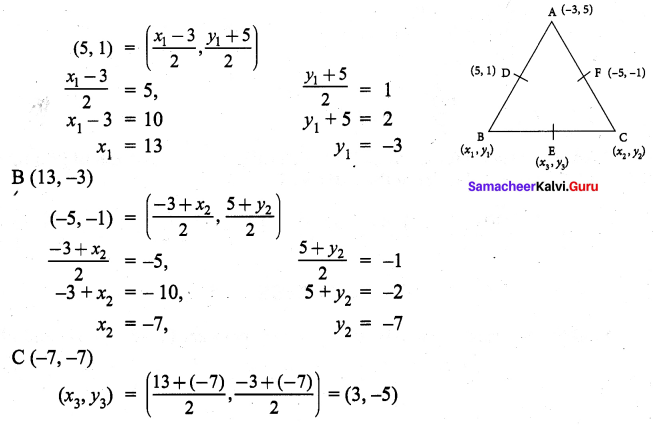Question 2.
If A(10, 11) and B(2, 3) are the coordinates of end points of diameter of circle. Then find the centre of the circle.
Solution: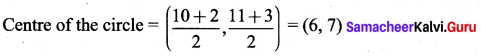Question 3.
Find the coordinates of the point which divides the line segment joining the points (3, 1) and (5, 13) internally in the ratio 3 : 5.
Solution: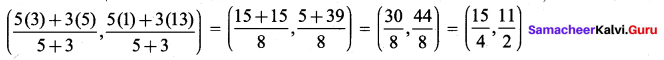Exercise 5.4

Question 1.
Using section formula, show that the points A(7, -5), B(9, -3) and C(13, 1) are collinear.
Solution: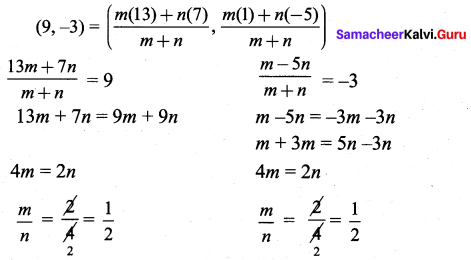Question 2.
A car travels at an uniform speed. At 2pm it is at a distance of 5 km at 6 pm it is at a distance of 120 km. Using section formula, find at what distance it will reach 2 mid night.
Solution: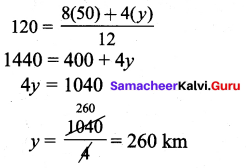Question 3.
Find the coordinates of the point which divides the line segment joining the point A(3, 7) and B(-11, -2) in the ratio 5 : 1.
Solution: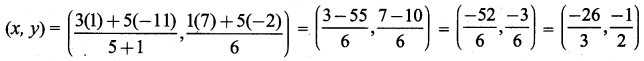Exercise 5.5

Question 1.
Find the centroid of the triangle whose vertices are (2, -5), (5, 11) and (9, 9)
Solution: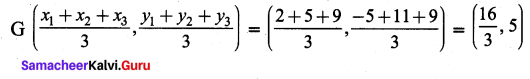Question 2.
If the centroid of a triangle is at (10, -1) and two of its vertices are (3, 2) and (5, -11). Find the third vertex of the triangle.
Solution: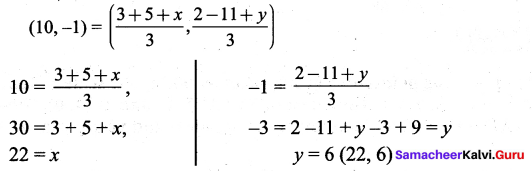Exercise 5.6

Multiple Choice Questions :

Question 1.
The point (-2, 7) lies is the quadrant
(1) I
(2) II
(3) III
(4) IV
Hint:
(-, +) lies in IInd quadrant
Solution:
(2) II

Question 2.
The point (x, 0) where x < 0 lies on
(1) OX
(2) OY
(3) OX’
(4) OY’
Hint:
(-, 0) lies on OX’
Solution:
(3) OX’

Question 3.
For a point A(a, b) lying in quadrant III.
(1) a > 0, b < 0
(2) a < 0, b < 0
(3) a > 0, b > 0
(4) a < 0, b > 0
Hint:
(-, -) lies in IIIrd quadrant
Solution:
(2) a < 0, b < 0

Question 4.
The diagonal of a square formed by the points (1, 0) (0, 1) and (-1, 0) is
(1) 2
(2) 4
(3) 2–√
(4) 8
Hint: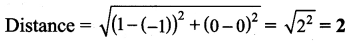Solution:
(1) 2

Question 5.
The triangle obtained by joining the points A(-5, 0) B(5, 0) and C(0, 6) is
(1) an isosceles triangle
(2) right triangle
(3) scalene triangle
(4) an equilateral triangle
Hint:
Triangles having two sides equal are called isosceles.
Solution:
(a) an isosceles triangle

Text Book Activities

Activity 1.
Plot the following points on a graph sheet by taking the scale as 1cm = 1 unit. Find how far the points are from each other? A (1, 0) and D (4, 0). Find AD and also DA. Is AD = DA? You plot another set of points and verify your result.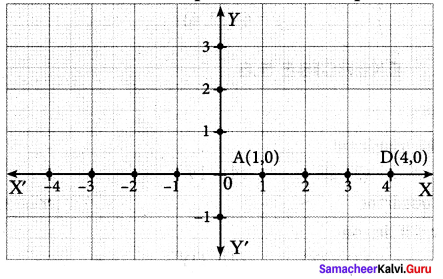Solution: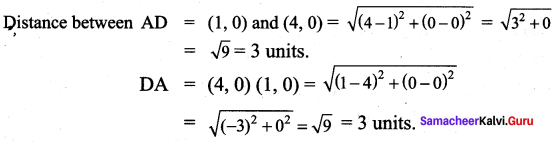## How to Prepare using Samacheer Kalvi 9th Maths Chapter 5 Coordinate Geometry Notes PDF?

Students must prepare for the upcoming exams from Samacheer Kalvi 9th Maths Chapter 5 Coordinate Geometry Notes PDF by following certain essential steps which are provided below.

• Use Samacheer Kalvi 9th Maths Chapter 5 Coordinate Geometry notes by paying attention to facts and ideas.
• Pay attention to the important topics
• Refer TN Board books as well as the books recommended.
• Correctly follow the notes to reduce the number of questions being answered in the exam incorrectly
• Highlight and explain the concepts in details.

## Frequently Asked Questions on Samacheer Kalvi 9th Maths Chapter 5 Coordinate Geometry Notes

#### How to use Samacheer Kalvi 9th Maths Chapter 5 Coordinate Geometry Notes for preparation??

Read TN Board thoroughly, make separate notes for points you forget, formulae, reactions, diagrams. Highlight important points in the book itself and make use of the space provided in the margin to jot down other important points on the same topic from different sources.

#### How to make notes for Samacheer Kalvi 9th Maths Chapter 5 Coordinate Geometry exam?

Read from hand-made notes prepared after understanding concepts, refrain from replicating from the textbook. Use highlighters for important points. Revise from these notes regularly and formulate your own tricks, shortcuts and mnemonics, mappings etc.
Share: